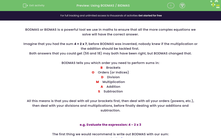# Using BODMAS / BIDMAS

In this worksheet, students will follow the rules of BODMAS / BIDMAS to prioritise the order of operations to successfully reach the correct answer in mental calculations.Key stage:  KS 4

Year:  GCSE

GCSE Subjects:   Maths

GCSE Boards:   Pearson Edexcel, OCR, Eduqas, AQA,

Curriculum topic:   Number, Number Operations and Integers

Curriculum subtopic:   Structure and Calculation Combining Arithmetic Operations

Difficulty level:#### Worksheet Overview

BODMAS or BIDMAS is a powerful tool we use in maths to ensure that all the more complex equations we solve will have the correct answer.

Imagine that you had the sum 4 + 2 x 7, before BODMAS was invented, nobody knew if the multiplication or the addition should be tackled first.

Both answers that you could get (56 and 18) may both have been right, but BODMAS changed that.

BODMAS tells you which order you need to perform sums in:

B    Brackets

O    Orders (or Indices)
D    Division
M    Multiplication
S    Subtraction

All this means is that you deal with all your brackets first, then deal with all your orders (powers, etc.), then deal with your divisions and multiplications, before finally dealing with your additions and subtraction.

e.g. Evaluate the expression: 4 - 2 x 3

The first thing we would recommend is write out BODMAS with our sum:

4 - 2 x 3

B           ----

O           ----

D           ----
M           4 - 6
A           ----
S          - 2

From this, you can see that we have ignored brackets, orders and division, before we have worked out the multiplication (2 x 3 = 6) and replaced this value in our original sum.

Then we ignored the addition and finally subtracted 6 from 4 at the end to reach an answer of -2.

e.g. Work out: (3 + 2)2 - (6 ÷ 2)

(3 + 2)2 - (6 ÷ 2)

B           (5)2 - (3)

O           25 - 3

D           ----
M           ----
A           ----
S           22

Once again, we have worked through each step in turn, ignoring the symbols that aren't applicable, working out one calculation at a time.

Now it's your turn to give this a try!

In this activity, you will follow the rules of BODMAS / BIDMAS to prioritise the order of operations so that you successfully reach the correct answer in mental calculations.

### What is EdPlace?

We're your National Curriculum aligned online education content provider helping each child succeed in English, maths and science from year 1 to GCSE. With an EdPlace account you’ll be able to track and measure progress, helping each child achieve their best. We build confidence and attainment by personalising each child’s learning at a level that suits them.

Get started